# Time of Concentration

In addition to manual entry, Hydrology Studio computes Time of Concentration using one of three methods,

1. Lag
2. Kirpich
3. TR55

## Lag Method

This is the TR-20 default method where: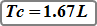Where:
L = Lag time (hrs)
l = Hydraulic length
S = (1000 / CN) – 10
Y = Average basin slope (%)
CN = Curve number

## Kirpich Method

Generally used for natural basins with well defined routes for overland flow along bare earth or mowed grass roadside channels. It is similar to the Lag method but will give shorter times compared to the Lag method.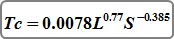Where:
Tc = Time of concentration (min)
L = Hydraulic length (ft)
S = Average basin slope (ft/ft)

## TR-55 Method

Tc is broken into 3-components or segments as prescribed by TR55. The final Tc is the sum total of the three components.

Slopes are inputted in percent in Hydrology Studio, not ft/ft as in TR55.

Tc = TSheet + TShallow + TChannel

### Sheet Flow Time

Flow over plane surfaces and typically ranges between 125 to 150 feet.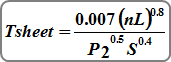Where:
n = Manning’s roughness coefficient
L = Flow Length (must be <=100 ft)
P2 = Two-year 24-hr rainfall (in)
S = Land Slope (ft/ft)

### Shallow Concentrated Flow Time

After about 100 feet, sheet flow becomes shallow concentrated flow. The computed Average Velocity described below is based on the solution of Manning’s equation with different assumptions for n (Manning’s roughness coefficient) and r (hydraulic radius, ft). Per TR55, for for paved areas, n is 0.025 and r is 0.2; unpaved areas, n is 0.05 and r is 0.4.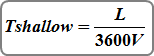Where:
L = Flow Length (ft)
V = Average velocity (ft/s) and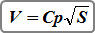Where:
Cp = 20.3282 paved surfaces
Cp = 16.1345 unpaved surfaces
S = Watercourse slope (ft/ft)

### Channel Flow Time

Occurs within channels, swales, ditches, streams or even piped systems. Manning’s equation is used to compute velocity.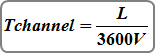Where:
L = Flow length (ft)
V = Average velocity (ft/s) and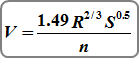Where:
V = Average velocity (ft/s)
R = Hydraulic radius (ft) = a/wp
S = Channel slope (ft/ft)
n = Manning’s roughness coefficient